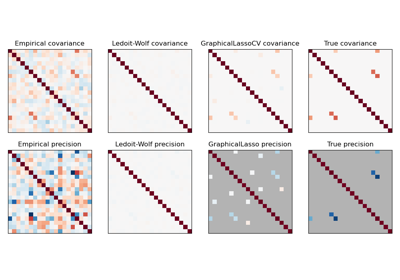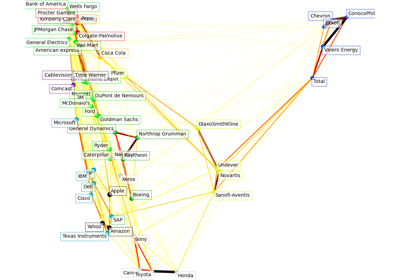# sklearn.covariance.GraphicalLassoCV¶

class sklearn.covariance.GraphicalLassoCV(*, alphas=4, n_refinements=4, cv=None, tol=0.0001, enet_tol=0.0001, max_iter=100, mode='cd', n_jobs=None, verbose=False, assume_centered=False)[source]

Sparse inverse covariance w/ cross-validated choice of the l1 penalty.

See glossary entry for cross-validation estimator.

Read more in the User Guide.

Changed in version v0.20: GraphLassoCV has been renamed to GraphicalLassoCV

Parameters:
alphasint or array-like of shape (n_alphas,), dtype=float, default=4

If an integer is given, it fixes the number of points on the grids of alpha to be used. If a list is given, it gives the grid to be used. See the notes in the class docstring for more details. Range is (0, inf] when floats given.

n_refinementsint, default=4

The number of times the grid is refined. Not used if explicit values of alphas are passed. Range is [1, inf).

cvint, cross-validation generator or iterable, default=None

Determines the cross-validation splitting strategy. Possible inputs for cv are:

• None, to use the default 5-fold cross-validation,

• integer, to specify the number of folds.

• An iterable yielding (train, test) splits as arrays of indices.

For integer/None inputs KFold is used.

Refer User Guide for the various cross-validation strategies that can be used here.

Changed in version 0.20: cv default value if None changed from 3-fold to 5-fold.

tolfloat, default=1e-4

The tolerance to declare convergence: if the dual gap goes below this value, iterations are stopped. Range is (0, inf].

enet_tolfloat, default=1e-4

The tolerance for the elastic net solver used to calculate the descent direction. This parameter controls the accuracy of the search direction for a given column update, not of the overall parameter estimate. Only used for mode=’cd’. Range is (0, inf].

max_iterint, default=100

Maximum number of iterations.

mode{‘cd’, ‘lars’}, default=’cd’

The Lasso solver to use: coordinate descent or LARS. Use LARS for very sparse underlying graphs, where number of features is greater than number of samples. Elsewhere prefer cd which is more numerically stable.

n_jobsint, default=None

Number of jobs to run in parallel. None means 1 unless in a joblib.parallel_backend context. -1 means using all processors. See Glossary for more details.

Changed in version v0.20: n_jobs default changed from 1 to None

verbosebool, default=False

If verbose is True, the objective function and duality gap are printed at each iteration.

assume_centeredbool, default=False

If True, data are not centered before computation. Useful when working with data whose mean is almost, but not exactly zero. If False, data are centered before computation.

Attributes:
location_ndarray of shape (n_features,)

Estimated location, i.e. the estimated mean.

covariance_ndarray of shape (n_features, n_features)

Estimated covariance matrix.

precision_ndarray of shape (n_features, n_features)

Estimated precision matrix (inverse covariance).

alpha_float

Penalization parameter selected.

cv_results_dict of ndarrays

A dict with keys:

alphasndarray of shape (n_alphas,)

All penalization parameters explored.

split(k)_test_scorendarray of shape (n_alphas,)

Log-likelihood score on left-out data across (k)th fold.

New in version 1.0.

mean_test_scorendarray of shape (n_alphas,)

Mean of scores over the folds.

New in version 1.0.

std_test_scorendarray of shape (n_alphas,)

Standard deviation of scores over the folds.

New in version 1.0.

split(k)_scorendarray of shape (n_alphas,)

Log-likelihood score on left-out data across (k)th fold.

Deprecated since version 1.0: split(k)_score is deprecated in 1.0 and will be removed in 1.2. Use split(k)_test_score instead.

mean_scorendarray of shape (n_alphas,)

Mean of scores over the folds.

Deprecated since version 1.0: mean_score is deprecated in 1.0 and will be removed in 1.2. Use mean_test_score instead.

std_scorendarray of shape (n_alphas,)

Standard deviation of scores over the folds.

Deprecated since version 1.0: std_score is deprecated in 1.0 and will be removed in 1.2. Use std_test_score instead.

New in version 0.24.

n_iter_int

Number of iterations run for the optimal alpha.

n_features_in_int

Number of features seen during fit.

New in version 0.24.

feature_names_in_ndarray of shape (n_features_in_,)

Names of features seen during fit. Defined only when X has feature names that are all strings.

New in version 1.0.

graphical_lasso

L1-penalized covariance estimator.

GraphicalLasso

Sparse inverse covariance estimation with an l1-penalized estimator.

Notes

The search for the optimal penalization parameter (alpha) is done on an iteratively refined grid: first the cross-validated scores on a grid are computed, then a new refined grid is centered around the maximum, and so on.

One of the challenges which is faced here is that the solvers can fail to converge to a well-conditioned estimate. The corresponding values of alpha then come out as missing values, but the optimum may be close to these missing values.

In fit, once the best parameter alpha is found through cross-validation, the model is fit again using the entire training set.

Examples

>>> import numpy as np
>>> from sklearn.covariance import GraphicalLassoCV
>>> true_cov = np.array([[0.8, 0.0, 0.2, 0.0],
...                      [0.0, 0.4, 0.0, 0.0],
...                      [0.2, 0.0, 0.3, 0.1],
...                      [0.0, 0.0, 0.1, 0.7]])
>>> np.random.seed(0)
>>> X = np.random.multivariate_normal(mean=[0, 0, 0, 0],
...                                   cov=true_cov,
...                                   size=200)
>>> cov = GraphicalLassoCV().fit(X)
>>> np.around(cov.covariance_, decimals=3)
array([[0.816, 0.051, 0.22 , 0.017],
[0.051, 0.364, 0.018, 0.036],
[0.22 , 0.018, 0.322, 0.094],
[0.017, 0.036, 0.094, 0.69 ]])
>>> np.around(cov.location_, decimals=3)
array([0.073, 0.04 , 0.038, 0.143])


Methods

 error_norm(comp_cov[, norm, scaling, squared]) Compute the Mean Squared Error between two covariance estimators. fit(X[, y]) Fit the GraphicalLasso covariance model to X. get_params([deep]) Get parameters for this estimator. Getter for the precision matrix. Compute the squared Mahalanobis distances of given observations. score(X_test[, y]) Compute the log-likelihood of X_test under the estimated Gaussian model. set_params(**params) Set the parameters of this estimator.
error_norm(comp_cov, norm='frobenius', scaling=True, squared=True)[source]

Compute the Mean Squared Error between two covariance estimators.

Parameters:
comp_covarray-like of shape (n_features, n_features)

The covariance to compare with.

norm{“frobenius”, “spectral”}, default=”frobenius”

The type of norm used to compute the error. Available error types: - ‘frobenius’ (default): sqrt(tr(A^t.A)) - ‘spectral’: sqrt(max(eigenvalues(A^t.A)) where A is the error (comp_cov - self.covariance_).

scalingbool, default=True

If True (default), the squared error norm is divided by n_features. If False, the squared error norm is not rescaled.

squaredbool, default=True

Whether to compute the squared error norm or the error norm. If True (default), the squared error norm is returned. If False, the error norm is returned.

Returns:
resultfloat

The Mean Squared Error (in the sense of the Frobenius norm) between self and comp_cov covariance estimators.

fit(X, y=None)[source]

Fit the GraphicalLasso covariance model to X.

Parameters:
Xarray-like of shape (n_samples, n_features)

Data from which to compute the covariance estimate.

yIgnored

Not used, present for API consistency by convention.

Returns:
selfobject

Returns the instance itself.

get_params(deep=True)[source]

Get parameters for this estimator.

Parameters:
deepbool, default=True

If True, will return the parameters for this estimator and contained subobjects that are estimators.

Returns:
paramsdict

Parameter names mapped to their values.

get_precision()[source]

Getter for the precision matrix.

Returns:
precision_array-like of shape (n_features, n_features)

The precision matrix associated to the current covariance object.

mahalanobis(X)[source]

Compute the squared Mahalanobis distances of given observations.

Parameters:
Xarray-like of shape (n_samples, n_features)

The observations, the Mahalanobis distances of the which we compute. Observations are assumed to be drawn from the same distribution than the data used in fit.

Returns:
distndarray of shape (n_samples,)

Squared Mahalanobis distances of the observations.

score(X_test, y=None)[source]

Compute the log-likelihood of X_test under the estimated Gaussian model.

The Gaussian model is defined by its mean and covariance matrix which are represented respectively by self.location_ and self.covariance_.

Parameters:
X_testarray-like of shape (n_samples, n_features)

Test data of which we compute the likelihood, where n_samples is the number of samples and n_features is the number of features. X_test is assumed to be drawn from the same distribution than the data used in fit (including centering).

yIgnored

Not used, present for API consistency by convention.

Returns:
resfloat

The log-likelihood of X_test with self.location_ and self.covariance_ as estimators of the Gaussian model mean and covariance matrix respectively.

set_params(**params)[source]

Set the parameters of this estimator.

The method works on simple estimators as well as on nested objects (such as Pipeline). The latter have parameters of the form <component>__<parameter> so that it’s possible to update each component of a nested object.

Parameters:
**paramsdict

Estimator parameters.

Returns:
selfestimator instance

Estimator instance.

## Examples using sklearn.covariance.GraphicalLassoCV¶Sparse inverse covariance estimation

Sparse inverse covariance estimationVisualizing the stock market structure

Visualizing the stock market structure